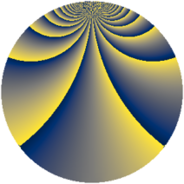# Properties

 Label 920.2.bbLevel $920$ Weight $2$ Character orbit 920.bb Rep. character $\chi_{920}(11,\cdot)$ Character field $\Q(\zeta_{22})$ Dimension $960$ Newform subspaces $2$ Sturm bound $288$ Trace bound $5$

# Related objects

## Defining parameters

 Level: $$N$$ $$=$$ $$920 = 2^{3} \cdot 5 \cdot 23$$ Weight: $$k$$ $$=$$ $$2$$ Character orbit: $$[\chi]$$ $$=$$ 920.bb (of order $$22$$ and degree $$10$$) Character conductor: $$\operatorname{cond}(\chi)$$ $$=$$ $$184$$ Character field: $$\Q(\zeta_{22})$$ Newform subspaces: $$2$$ Sturm bound: $$288$$ Trace bound: $$5$$ Distinguishing $$T_p$$: $$7$$

## Dimensions

The following table gives the dimensions of various subspaces of $$M_{2}(920, [\chi])$$.

Total New Old
Modular forms 1480 960 520
Cusp forms 1400 960 440
Eisenstein series 80 0 80

## Trace form

 $$960q + 4q^{2} - 8q^{4} + 10q^{6} - 2q^{8} - 96q^{9} + O(q^{10})$$ $$960q + 4q^{2} - 8q^{4} + 10q^{6} - 2q^{8} - 96q^{9} + 6q^{12} - 24q^{16} + 14q^{18} + 4q^{24} - 96q^{25} + 14q^{26} + 24q^{27} - 16q^{32} - 22q^{34} + 108q^{36} - 110q^{38} + 88q^{40} + 22q^{44} - 152q^{46} + 110q^{48} - 96q^{49} - 18q^{50} - 14q^{52} - 54q^{54} + 110q^{56} - 80q^{58} - 24q^{59} + 22q^{60} + 30q^{62} - 14q^{64} + 6q^{72} - 22q^{74} - 182q^{78} - 112q^{81} - 118q^{82} - 308q^{84} - 176q^{88} - 130q^{92} - 148q^{94} + 130q^{96} - 196q^{98} + O(q^{100})$$

## Decomposition of $$S_{2}^{\mathrm{new}}(920, [\chi])$$ into newform subspaces

Label Dim. $$A$$ Field CM Traces $q$-expansion
$$a_2$$ $$a_3$$ $$a_5$$ $$a_7$$
920.2.bb.a $$480$$ $$7.346$$ None $$2$$ $$0$$ $$-48$$ $$0$$
920.2.bb.b $$480$$ $$7.346$$ None $$2$$ $$0$$ $$48$$ $$0$$

## Decomposition of $$S_{2}^{\mathrm{old}}(920, [\chi])$$ into lower level spaces

$$S_{2}^{\mathrm{old}}(920, [\chi]) \cong$$ $$S_{2}^{\mathrm{new}}(184, [\chi])$$$$^{\oplus 2}$$